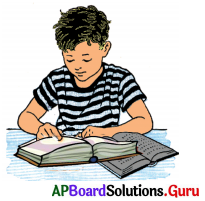SCERT AP 7th Class Maths Solutions Pdf Chapter 2 Fractions, Decimals and Rational Numbers Ex 2.2 Textbook Exercise Questions and Answers.

## AP State Syllabus 7th Class Maths Solutions 2nd Lesson Fractions, Decimals and Rational Numbers Exercise 2.2

Question 1.
Find the product of the following.
(i) 23.4 × 6
= $$\frac{234}{10} \times \frac{6}{1}$$
= $$\frac{1404}{10}$$
∴ 23.4 × 6 = 140.4

(ii) 681.25 × 9
681.25 × 9
= $$\frac{68125}{100} \times \frac{9}{1}$$
= $$\frac{68125 \times 9}{100}$$
= $$\frac{613125}{100}$$
∴ 681.25 × 9
= 6131.25

(iii) 53.29 × 14
53.29 × 14
= $$\frac{5329}{100} \times \frac{14}{1}$$
= $$\frac{74606}{100}$$
∴ 53.29 × 14
= 746.06

(iv) 8 × 2.52
8 × 2.52
= 8 × $$\frac{252}{100}$$
= $$\frac{8 \times 252}{100}$$
= $$\frac{2016}{100}$$
∴ 8 × 2.52 = 20.16

(v) 25 × 2.013
25 × 2.013
= 25 × $$\frac{2013}{1000}$$
= $$\frac{25 \times 2013}{1000}$$
= $$\frac{50325}{1000}$$
∴ 25 × 2.013 = 50.325Question 2.
Fill the blanks in the table.

 Multiplications Product 36.21 × 10 362.1 23.104 × 100 2310.4 6.24 × 1000 6240.0 0.02105 × 1000 21.05 9.234 × 100 923.4 1.3004 × 1000 1300.4 5.9001 × 10 59.001

Question 3.
Find the product.
(i) 5.1 × 8.1
= $$\frac{51}{10} \times \frac{81}{10}$$
= $$\frac{51 \times 81}{10 \times 10}$$
= $$\frac{4131}{100}$$
∴ 5.1 × 8.1 = 41.31

(ii) 63.205 × 0.27
63.205 × 0.27
= $$\frac{63205}{1000} \times \frac{27}{100}$$
= $$\frac{63205 \times 27}{1000 \times 100}$$
= $$\frac{1706535}{100000}$$
∴ 63.205 × 0.27 = 17.06535

(iii) 1.321 × 0.9
1.321 × 0.9
= $$\frac{1321}{1000} \times \frac{9}{10}$$
= $$\frac{1321 \times 9}{1000 \times 10}$$
= $$\frac{11889}{10000}$$
∴ 1.321 × 0.9 = 1.1889

(iv) 6.51 × 0.99
6.51 × 0.99
= $$\frac{651}{100} \times \frac{99}{100}$$
= $$\frac{651 \times 99}{100 \times 100}$$
= $$\frac{64449}{10000}$$
∴ 6.51 × 0.99 = 6.4449

(v) 837.6 × 0.006
837.6 × 0.006
= $$\frac{8376}{10} \times \frac{6}{1000}$$
= $$\frac{8376 \times 6}{10 \times 1000}$$
= $$\frac{50256}{10000}$$
∴ 837.6 × 0.006 = 5.0256

Question 4.
Rithesh reads a book for 2.5 hours everyday. If he reads the entire book in a week, then how many hours all together are required for him to read the book ?Given, time taken to read a book per day = 2.5 hours.

Time taken to read entire book in a week (one week = 7 days)
= 2.5 × 7 days
= $$\frac{25}{10}$$ × 7
= $$\frac{175}{10}$$
∴ Time taken to read entire book = 17.5 hours.Question 5.
Find the area of the rectangle whose length and breadth are 5.3 cm, 2.7 cm respectively.
Given length of the rectangle l = 5.3 cm
Breadth of the rectangle b = 2.7 cm
Area of the rectangle = l × b
= 5.3 × 2.7
= $$\frac{53}{10} \times \frac{27}{10}$$
= $$\frac{53 \times 27}{10 \times 10}$$
∴ Area of the rectangle = $$\frac{1431}{100}$$
= 14.31 sq.cm

Question 6.
If the cost of each cement bag is ₹ 326.50, then find the cost of 24 bags of cement.
Given, cost of each cement bag
= ₹ 326.50

Cost of 24 cement bags
= 326.50 × 24
= $$\frac{32650}{100}$$ × 24
= $$\frac{32650 \times 24}{100}$$
= $$\frac{783600}{100}$$

∴ Cost of 24 cement bags = ₹ 7,836.

Question 7.
Dharmika purchased chudidhar material of 1.40 m at the rate of 7152.5 pet irtetre. Find the amount to be paid.
Given cost of one meter chudidhar material = 7152.50
Cost of 1.40 meter chudidhar material = 152.50 × 1.40
= $$\frac{15250}{100} \times \frac{140}{100}$$
= $$\frac{15250 \times 140}{100 \times 100}$$
= $$\frac{2135000}{10000}$$
∴ Cost of 1.40 meter chudidhar material = ₹ 213.50

Question 8.
If a picture chart costs 74.25. Amrutha wants to buy 16 charts to make an album. How much money does she has to pay ?
= $$\frac{425}{100}$$ × 16
= $$\frac{425 \times 16}{100}$$
∴ Cost of 16 charts to make album = $$\frac{6800}{100}$$ = ₹ 68